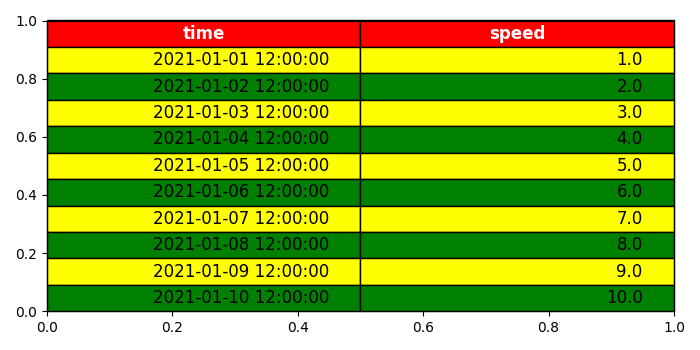# How can I place a table on a plot in Matplotlib?

MatplotlibPythonData Visualization

## Steps

• Using the subplots() method, create a figure and a set of subplots with figure size (7, 7).

• Create a data frame with two keys, time and speed.

• Get the size of the array.

• Add a table to the current axis using the table method.

• Shrink the font size until the text fits into the cell width.

• Set the font size in the table.

• Set the face color, edge color, and text color by iterating the matplotlib table.

• Save and display the figure.

## Example

import numpy as np
import pandas as pd
from matplotlib import pyplot as plt
plt.rcParams["figure.figsize"] = [7.00, 3.50]
plt.rcParams["figure.autolayout"] = True
fig, ax = plt.subplots()
df = pd.DataFrame(dict(time=list(pd.date_range("2021-01-01 12:00:00",
periods=10)), speed=np.linspace(1, 10, 10)))
size = (np.array(df.shape[::-1]) + np.array([0, 1])) * np.array([3.0, 6.0])
mpl_table = ax.table(cellText=df.values, bbox=[0, 0, 1, 1], colLabels=df.columns)
mpl_table.auto_set_font_size(False)
mpl_table.set_fontsize(12)
for k, cell in mpl_table._cells.items():
cell.set_edgecolor('black')
if k == 0 or k < 0:
cell.set_text_props(weight='bold', color='w')
cell.set_facecolor('red')
else:
cell.set_facecolor(['green', 'yellow'][k % 2])
plt.show()

## Output